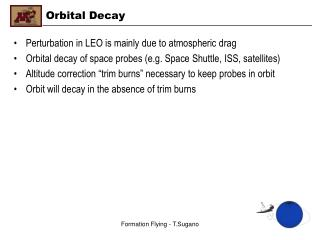DownloadDownload PresentationOrbital Decay

# Orbital Decay

Télécharger la présentation## Orbital Decay

- - - - - - - - - - - - - - - - - - - - - - - - - - - E N D - - - - - - - - - - - - - - - - - - - - - - - - - - -
##### Presentation Transcript

1. Orbital Decay • Perturbation in LEO is mainly due to atmospheric drag • Orbital decay of space probes (e.g. Space Shuttle, ISS, satellites) • Altitude correction “trim burns” necessary to keep probes in orbit • Orbit will decay in the absence of trim burns Formation Flying - T.Sugano

2. Orbit Lifetime Estimation • Estimation of the orbit lifetime of our satellite after mission • Consider atmospheric drag effect only • Mission orbit is assumed virtually circular for simplicity Formation Flying - T.Sugano

3. Orbit Lifetime Equation • Circular Orbit Lifetime Equation (Approximation) a0 = initial altitude S = projected area of the space probe m = space probe mass Formation Flying - T.Sugano

4. Exponential Atmospheric Model • Scale height, H, obtained from tabulated data Formation Flying - T.Sugano

5. Assumptions set forth for our lifetime computation • Assumptions: (Made for worst case or shortest decay) m = 50 kg (maximum); S = 0.385m2 (spherical correction of max volume) CD = 3.0 (upper bound value in LEO probes) a0 = 6400 + 300 km (typical altitude for STS or ISS) Δ = 150 – 300 = - 150 km (typical re-entry altitude, note the minus sign) f = 1 (ignore latitude effect; not significant (<10%)) ρ0 = 2.418x10-11 kg/m3 (Table, 300 km base altitude) • Unavoidable uncertainty  Scale height, H - Not constant between orbit and re-entry altitude - Take H = 30 km, so β = 1 / (30 km) Formation Flying - T.Sugano

6. Computation Result • Based on the assumptions we made - T = tau_0 * 189.565 - T = (approx. 1.5 hr of initial orbit period)*(190) = 12 days • LEO Nanosat at 300 km of altitude will take 12 days to decay. Formation Flying - T.Sugano

7. Conclusion • Our Nanosat does not decease for 12 days • Retroburn delta-V input to decelerate the Nanosat for faster decay will be costly without a compelling space debris concern(?) • Unless allowed to dispose of the Nanosat in space, retrieval is rather recommended(?) • Retrieval may be attained fairly easily by using robot arm of STS perhaps equipped with capture net(?) Formation Flying - T.Sugano